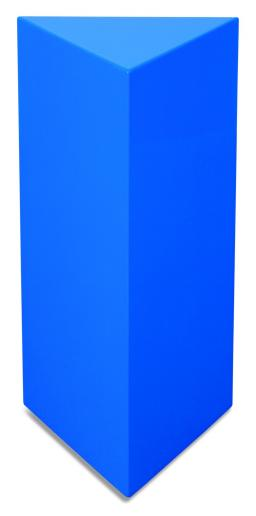# Prism-shaped 6137

The prism-shaped vessel with a rhomboid base has one base diagonal of 10 cm and the edge of the base 14 cm.
The edge of the base and the prism height are in a ratio of 2:5.
How many liters of water is in the container when it is filled to four-fifths of the volume?

V =  3.6615 l

### Step-by-step explanation:

Try calculation via our triangle calculator.Did you find an error or inaccuracy? Feel free to write us. Thank you!

Tips for related online calculators
Need help calculating sum, simplifying, or multiplying fractions? Try our fraction calculator.
Do you know the volume and unit volume, and want to convert volume units?